Courses

# Circuit Theorems - 1

## 10 Questions MCQ Test Topicwise Question Bank for Electrical Engineering | Circuit Theorems - 1

Description
This mock test of Circuit Theorems - 1 for Electrical Engineering (EE) helps you for every Electrical Engineering (EE) entrance exam. This contains 10 Multiple Choice Questions for Electrical Engineering (EE) Circuit Theorems - 1 (mcq) to study with solutions a complete question bank. The solved questions answers in this Circuit Theorems - 1 quiz give you a good mix of easy questions and tough questions. Electrical Engineering (EE) students definitely take this Circuit Theorems - 1 exercise for a better result in the exam. You can find other Circuit Theorems - 1 extra questions, long questions & short questions for Electrical Engineering (EE) on EduRev as well by searching above.
QUESTION: 1

Solution:
QUESTION: 2

### In any lumped network with elements in b  branches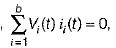for all holds good  according to

Solution: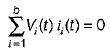means the total sum of instantenous power consumed in various elements in the branches of a network is zero, which is the statement of Tellegen’s theorem.

QUESTION: 3

### The Theven's equivalent resistance to the left of AB in figure given below is given by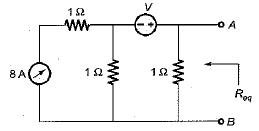Solution:

For finding the Thevenin’s equivalent resistance, 8 A current source will be open circuited while v volt voltage source will be short circuited.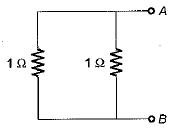Hence,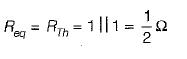QUESTION: 4

For which of the following, Thevenin’s and Norton’s equivalents can’t be developed?

Solution:
QUESTION: 5

The value of Z22 (Ω) for the circuit shown below is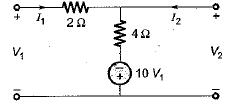Solution:

Applying KVL, we get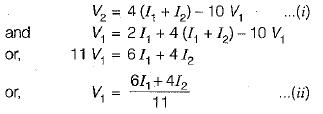Putting the value of V1 from equation (ii) into equation (i), we get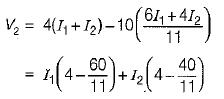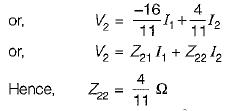QUESTION: 6

In which of the following it is not desired to attain the condition of maximum power transfer?

Solution:
QUESTION: 7

A simple equivalent circuit of the two terminal network shown in figure below is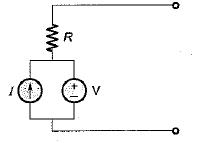Solution:

For the given circuit, Req across the given terminals = R.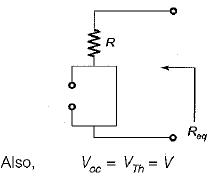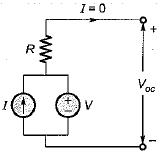QUESTION: 8

For the circuit shown below, a resistance R is to be connected across an active network, it is given that when

(i) R =∞, V = 5 volts

(ii) R = 0, current output of the active source is 2.5 A.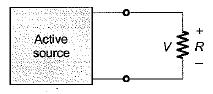What would be the current through tfwhen the value of R is 5 Ω?

Solution:

When R = ∞, Voc= V = 5 volts
When R = 0 , ISC = 2 .5 A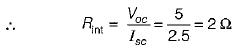Hence, the Thevenin's equivalent circuit will be drawn as shown below.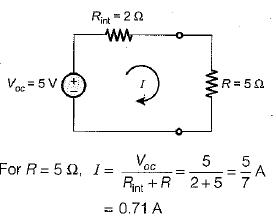QUESTION: 9

A generator of internal impedance I ZgI delivers maximum power to a load impedance Zp , only if

Solution:
QUESTION: 10

In the circuit shown below, the current through the 5Ω resistor is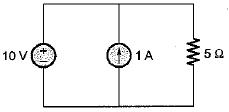Solution:

An ideal voltage source is never short circuited. Hence, we should not use superposition theorem. The voltage across the 5Ω resistor will be 10 volts. Hence, current through the 5 Ω resistor

=10/5 = 2 A